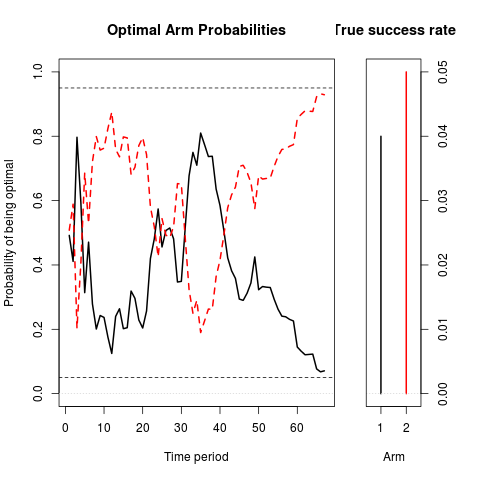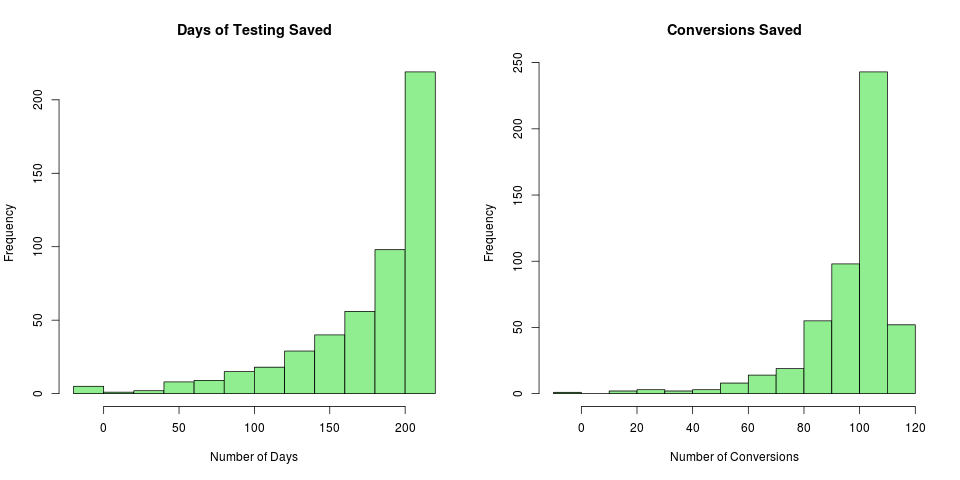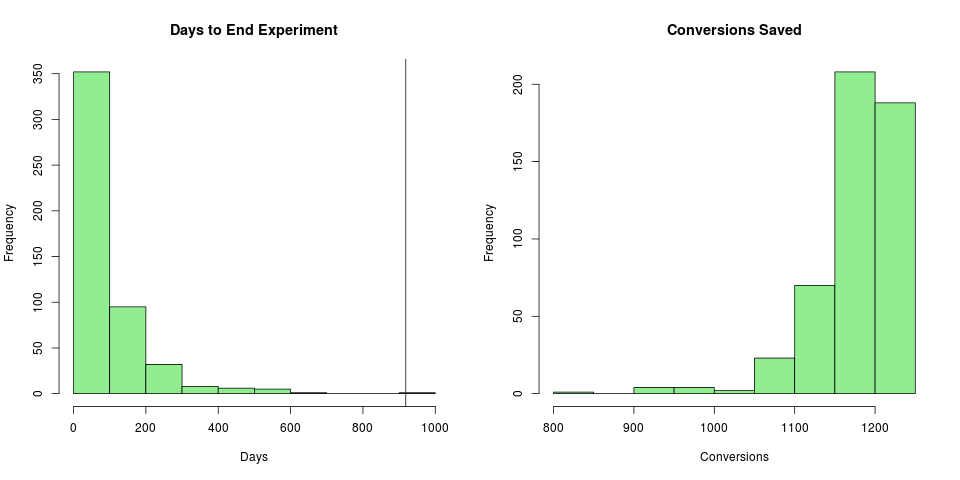# Multi-armed bandit experiments

By Steven L. Scott, PhD, Sr. Economic Analyst

This article describes the statistical engine behind Analytics Content Experiments. Analytics uses a multi-armed bandit approach to managing online experiments. A multi-armed bandit is a type of experiment where:

• The goal is to find the best or most profitable action
• The randomization distribution can be updated as the experiment progresses

The name "multi-armed bandit" describes a hypothetical experiment where you face several slot machines ("one-armed bandits") with potentially different expected payouts. You want to find the slot machine with the best payout rate, but you also want to maximize your winnings. The fundamental tension is between "exploiting" arms that have performed well in the past and "exploring" new or seemingly inferior arms in case they might perform even better. There are highly developed mathematical models for managing the bandit problem, which we use in Analytics content experiments.

This document starts with some general background on the use of multi-armed bandits in Analytics. Then it presents two examples of simulated experiments run using our multi-armed bandit algorithm. It then address some frequently asked questions, and concludes with an appendix describing technical computational and theoretical details.

### Background

#### How bandits work

Twice per day, we take a fresh look at your experiment to see how each of the variations has performed, and we adjust the fraction of traffic that each variation will receive going forward. A variation that appears to be doing well gets more traffic, and a variation that is clearly underperforming gets less. The adjustments we make are based on a statistical formula (see the appendix if you want details) that considers sample size and performance metrics together, so we can be confident that we’re adjusting for real performance differences and not just random chance. As the experiment progresses, we learn more and more about the relative payoffs, and so do a better job in choosing good variations.

#### Benefits

Experiments based on multi-armed bandits are typically much more efficient than "classical" A-B experiments based on statistical-hypothesis testing. They’re just as statistically valid, and in many circumstances they can produce answers far more quickly. They’re more efficient because they move traffic towards winning variations gradually, instead of forcing you to wait for a "final answer" at the end of an experiment. They’re faster because samples that would have gone to obviously inferior variations can be assigned to potential winners. The extra data collected on the high-performing variations can help separate the "good" arms from the "best" ones more quickly.

Basically, bandits make experiments more efficient, so you can try more of them. You can also allocate a larger fraction of your traffic to your experiments, because traffic will be automatically steered to better performing pages.

### Examples

#### A simple A/B test

Suppose you’ve got a conversion rate of 4% on your site. You experiment with a new version of the site that actually generates conversions 5% of the time. You don’t know the true conversion rates of course, which is why you’re experimenting, but let’s suppose you’d like your experiment to be able to detect a 5% conversion rate as statistically significant with 95% probability. A standard power calculation1 tells you that you need 22,330 observations (11,165 in each arm) to have a 95% chance of detecting a .04 to .05 shift in conversion rates. Suppose you get 100 sessions per day to the experiment, so the experiment will take 223 days to complete. In a standard experiment you wait 223 days, run the hypothesis test, and get your answer.

Now let’s manage the 100 sessions each day through the multi-armed bandit. On the first day about 50 sessions are assigned to each arm, and we look at the results. We use Bayes' theorem to compute the probability that the variation is better than the original2. One minus this number is the probability that the original is better. Let’s suppose the original got really lucky on the first day, and it appears to have a 70% chance of being superior. Then we assign it 70% of the traffic on the second day, and the variation gets 30%. At the end of the second day we accumulate all the traffic we’ve seen so far (over both days), and recompute the probability that each arm is best. That gives us the serving weights for day 3. We repeat this process until a set of stopping rules has been satisfied (we’ll say more about stopping rules below).

Figure 1 shows a simulation of what can happen with this setup. In it, you can see the serving weights for the original (the black line) and the variation (the red dotted line), essentially alternating back and forth until the variation eventually crosses the line of 95% confidence. (The two percentages must add to 100%, so when one goes up the other goes down). The experiment finished in 66 days, so it saved you 157 days of testing.Figure 1. A simulation of the optimal arm probabilities for a simple two-armed experiment. These weights give the fraction of the traffic allocated to each arm on each day.

Of course this is just one example. We re-ran the simulation 500 times to see how well the bandit fares in repeated sampling. The distribution of results is shown in Figure 2. On average the test ended 175 days sooner than the classical test based on the power calculation. The average savings was 97.5 conversions.Figure 2. The distributions of the amount of time saved and the number of conversions saved vs. a classical experiment planned by a power calculation. Assumes an original with 4% CvR and a variation with 5% CvR.

But what about statistical validity? If we’re using less data, doesn’t that mean we’re increasing the error rate? Not really. Out of the 500 experiments shown above, the bandit found the correct arm in 482 of them. That’s 96.4%, which is about the same error rate as the classical test. There were a few experiments where the bandit actually took longer than the power analysis suggested, but only in about 1% of the cases (5 out of 500).

We also ran the opposite experiment, where the original had a 5% success rate and the the variation had 4%. The results were essentially symmetric. Again the bandit found the correct arm 482 times out of 500. The average time saved relative to the classical experiment was 171.8 days, and the average number of conversions saved was 98.7.

#### Stopping the experiment

By default, we force the bandit to run for at least two weeks. After that, we keep track of two metrics.

The first is the probability that each variation beats the original. If we’re 95% sure that a variation beats the original then Analytics declares that a winner has been found. Both the two-week minimum duration and the 95% confidence level can be adjusted by the user.

The second metric that we monitor is is the "potential value remaining in the experiment", which is particularly useful when there are multiple arms. At any point in the experiment there is a "champion" arm believed to be the best. If the experiment ended "now", the champion is the arm you would choose. The "value remaining" in an experiment is the amount of increased conversion rate you could get by switching away from the champion. The whole point of experimenting is to search for this value. If you’re 100% sure that the champion is the best arm, then there is no value remaining in the experiment, and thus no point in experimenting. But if you’re only 70% sure that an arm is optimal, then there is a 30% chance that another arm is better, and we can use Bayes’ rule to work out the distribution of how much better it is. (See the appendix for computational details).

Analytics ends the experiment when there’s at least a 95% probability that the value remaining in the experiment is less than 1% of the champion’s conversion rate. That’s a 1% improvement, not a one percentage point improvement. So if the best arm has a conversion rate of 4%, then we end the experiment if the value remaining in the experiment is less than .04 percentage points of CvR.

Ending an experiment based on the potential value remaining is nice because it handles ties well. For example, in an experiment with many arms, it can happen that two or more arms perform about the same, so it does not matter which is chosen. You wouldn’t want to run the experiment until you found the optimal arm (because there are two optimal arms). You just want to run the experiment until you’re sure that switching arms won’t help you very much.

#### More complex experiments

The multi-armed bandit’s edge over classical experiments increases as the experiments get more complicated. You probably have more than one idea for how to improve your web page, so you probably have more than one variation that you’d like to test. Let’s assume you have 5 variations plus the original. You’re going to do a calculation where you compare the original to the largest variation, so we need to do some sort of adjustment to account for multiple comparisons. The Bonferroni correction is an easy (if somewhat conservative) adjustment, which can be implemented by dividing the significance level of the hypothesis test by the number of arms. Thus we do the standard power calculation with a significance level of .05 / (6 - 1), and find that we need 15,307 observations in each arm of the experiment. With 6 arms that’s a total of 91,842 observations. At 100 sessions per day the experiment would have to run for 919 days (over two and a half years). In real life it usually wouldn’t make sense to run an experiment for that long, but we can still do the thought experiment as a simulation.

Now let’s run the 6-arm experiment through the bandit simulator. Again, we will assume an original arm with a 4% conversion rate, and an optimal arm with a 5% conversion rate. The other 4 arms include one suboptimal arm that beats the original with conversion rate of 4.5%, and three inferior arms with rates of 3%, 2%, and 3.5%. Figure 3 shows the distribution of results. The average experiment duration is 88 days (vs. 919 days for the classical experiment), and the average number of saved conversions is 1,173. There is a long tail to the distribution of experiment durations (they don’t always end quickly), but even in the worst cases, running the experiment as a bandit saved over 800 conversions relative to the classical experiment.Figure 3. Savings from a six-armed experiment, relative to a Bonferroni adjusted power calculation for a classical experiment. The left panel shows the number of days required to end the experiment, with the vertical line showing the time required by the classical power calculation. The right panel shows the number of conversions that were saved by the bandit.

The cost savings are partially attributable to ending the experiment more quickly, and partly attributable to the experiment being less wasteful while it is running. Figure 4 shows the history of the serving weights for all the arms in the first of our 500 simulation runs. There is some early confusion as the bandit sorts out which arms perform well and which do not, but the very poorly performing arms are heavily downweighted very quickly. In this case, the original arm has a "lucky run" to begin the experiment, so it survives longer than some other competing arms. But after about 50 days, things have settled down into a two-horse race between the original and the ultimate winner. Once the other arms are effectively eliminated, the original and the ultimate winner split the 100 observations per day between them. Notice how the bandit is allocating observations efficiently from an economic standpoint (they’re flowing to the arms most likely to give a good return), as well as from a statistical standpoint (they’re flowing to the arms that we most want to learn about).